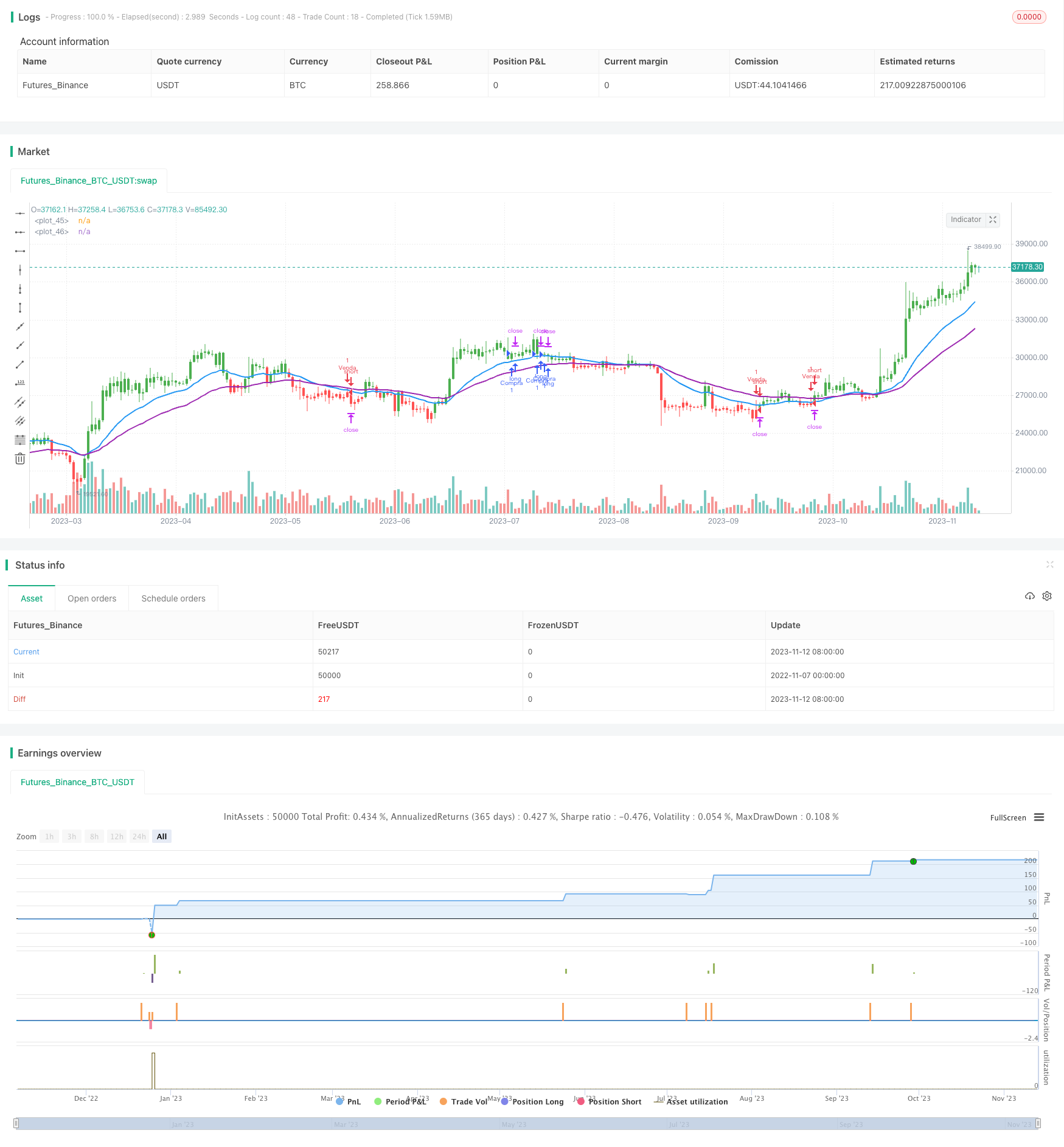# McGinley Moving Average Trading Strategy

Author: ChaoZhang, Date: 2023-11-14 15:48:46
Tags:## Overview

This strategy is based on the McGinley Dynamic Moving Average indicator. The McGinley MA indicator is an improved version of moving averages that can track price trends better. The strategy utilizes the signals generated by the McGinley MA indicator combined with price breakouts of MAs to establish trading rules for profit.

## Strategy Logic

The strategy mainly uses two moving averages, a 21-period EMA and a 42-period EMA. When the shorter MA crosses above the longer MA, it is considered a buy signal. When the shorter MA crosses below the longer MA, it is considered a sell signal.

In addition, the strategy also requires the price to be above the McGinley Dynamic MA and break above the shorter MA to generate a buy signal. For the sell signal, it also needs the price to be below the McGinley MA and break below the shorter MA.

Specifically, the buy signal is triggered when: shorter MA crosses above longer MA, close price above McGinley MA, close price breaks below shorter MA. The sell signal is triggered when: shorter MA crosses below longer MA, close price below McGinley MA, close price breaks above shorter MA.

The McGinley MA is calculated as: MDIt = MDIt-1 + (Close - MDIt-1) / Max(k * Period * (Close / MDIt-1)^4, 1). Where MDIt is the current value, MDIt-1 is previous value, Close is the closing price, k is the smoothing constant, and Period is the calculation period. This formula allows the MA to track price changes in real time.

1. McGinley MA improves the lagging issue of traditional MAs and can quickly capture trend changes.

2. Using dual MAs to generate signals can effectively filter false breakouts.

3. Adding price above/below McGinley MA avoids excessive trading in range-bound markets.

4. Using EMA makes the MA more sensitive to recent price changes.

## Risks

1. Whipsaws may generate false signals in sideways markets, causing losses. Parameters can be adjusted to filter signals.

2. Gap openings may fail to trigger entry in time. Entry rules could be relaxed.

3. Inadequate parameter tuning can impact strategy performance. Parameters should be optimized.

4. Long holding periods incur systemic risks. Consider using stop loss.

## Enhancements

1. Test different MA lengths to find optimal combinations.

2. Add other indicators like KD, MACD to improve entry/exit timing.

3. Adjust k value based on different products and markets to optimize McGinley MA calculation.

4. Incorporate volatility measures for dynamical position sizing and risk control.

5. Set stop loss to control losses. Trailing stop can also be tested to lock in profits.

## Conclusion

This strategy utilizes the fast-tracking ability of McGinley MA combined with price breakout signals to effectively follow trends and switch positions when trend reverses. Compared to traditional dual MA strategies, it can capture trend changes faster. However, risks exist that require optimization and tuning of parameters to control risks.

```/*backtest
start: 2022-11-07 00:00:00
end: 2023-11-13 00:00:00
period: 1d
basePeriod: 1h
exchanges: [{"eid":"Futures_Binance","currency":"BTC_USDT"}]
*/

// This source code is subject to the terms of the Mozilla Public License 2.0 at https://mozilla.org/MPL/2.0/

//@version=4
strategy(shorttitle="Maguila", title="McGinley Dynamic Indicator", overlay=true)

//Médias móveis
MA1Period=input(21, title="MA1")
MA2Period=input(42, title="MA2")

MA1 = ema(close, MA1Period)
MA2 = ema(close, MA2Period)

aboveAverage = MA1 >= MA2
hunderAverage = MA2 >= MA1

//Período do backtest
startDate = input(title="Start Date", type=input.integer, defval=28, minval=1, maxval=31)
startMonth = input(title="Start Month", type=input.integer, defval=5, minval=1, maxval=12)
startYear = input(title="Start Year", type=input.integer, defval=2019, minval=1800, maxval=2100)

endDate = input(title="End Date", type=input.integer, defval=28, minval=1, maxval=31)
endMonth = input(title="End Month", type=input.integer, defval=5, minval=1, maxval=12)
endYear = input(title="End Year", type=input.integer, defval=2030, minval=1800, maxval=2100)

//Verifica se o candle está dentro do período do backtest
inDateRange = (time >= timestamp(syminfo.timezone, startYear, startMonth, startDate, 0, 0)) and (time < timestamp(syminfo.timezone, endYear, endMonth, endDate, 0, 0))

//Número de periodos da média móvel
period  = input(title="Períodos", type=input.integer, defval=20)
//Constante K (0.6)
k = input(title="Constante K", type=input.float, defval=0.6)
//Preço de fechamento
closePrice = input(title="Preço", type=input.source, defval=close)

mdi = 0.0

//Fórmula de McGinley
mdi := na(mdi) ? closePrice : mdi + (closePrice - mdi) / max((k * period * pow(closePrice / mdi, 4)), 1)

//Regra de coloração
mdiColor = closePrice > mdi ? color.green : closePrice < mdi ? color.red : color.black

//Inserindo as informações no gráfico
plot(MA1, color=color.blue, linewidth=2)
plot(MA2, color=color.purple, linewidth=2)

barcolor(mdiColor)

//Estratégia
buySignal = aboveAverage and closePrice > mdi and crossunder(low, MA1) and close > MA1
buyLoss = closePrice < mdi and close < MA1 and close < MA2

if (inDateRange)
strategy.exit("Gain da compra", "Compra", qty=1, profit=20)
strategy.close("Compra", qty=1, when= buyLoss, comment="Loss na operação")

sellSignal = hunderAverage and closePrice < mdi and crossover(high, MA1) and close < MA1
sellLoss = closePrice > mdi and close > MA1 and close > MA2

if (inDateRange)
strategy.entry("Venda", strategy.short, qty=1, when= sellSignal)
strategy.exit("Gain da venda", "Venda", qty=1, profit=20)
strategy.close("Venda", qty=1, when= sellLoss, comment="Loss na operação")

if (not inDateRange)
strategy.close_all()

```

More